# Sparkfun COM-11408 4 Digit 7-Segment Display Hookup Guide

Have you ever wondered about how a 7-Segment LED actually works? This is a basic tutorial for using these inexpensive 4 digit displays.

BeginnerProtip3 hours9,214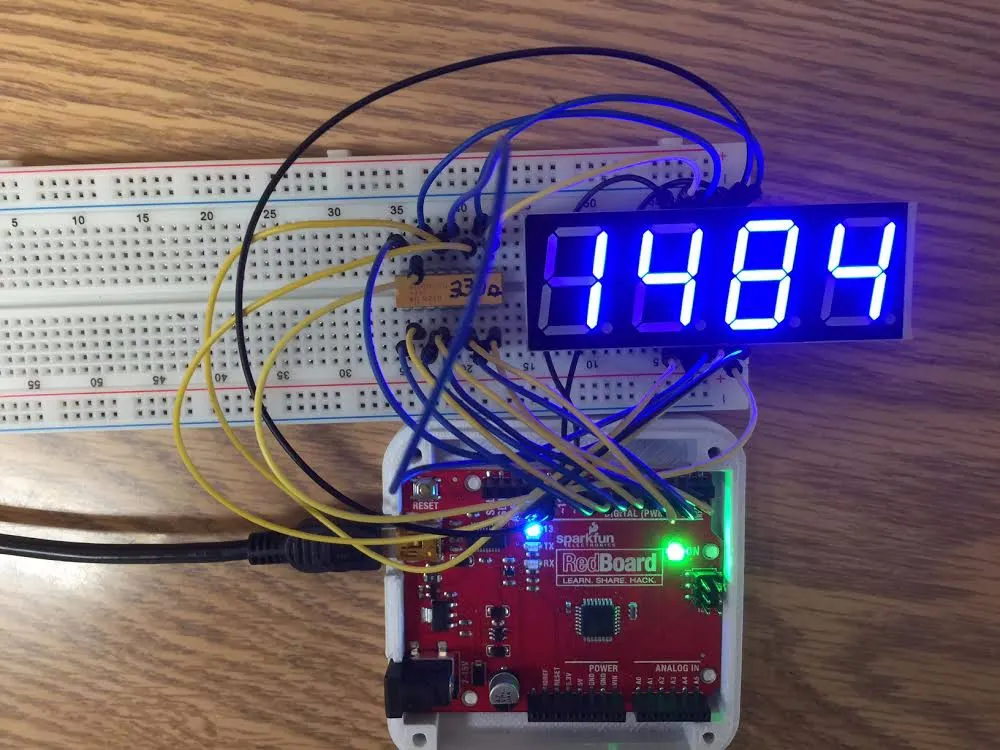## Things used in this project

### Hardware components

 SparkFun 4 Digit 7 Segment LED Display Manufacturer's part number ATA8401AB
×1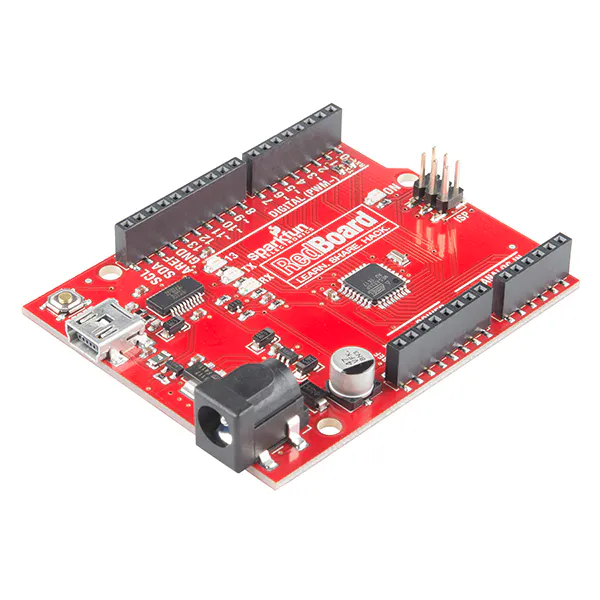SparkFun RedBoard Almost any Arduino compatible with 12 or more digital I/O pins can be used
×1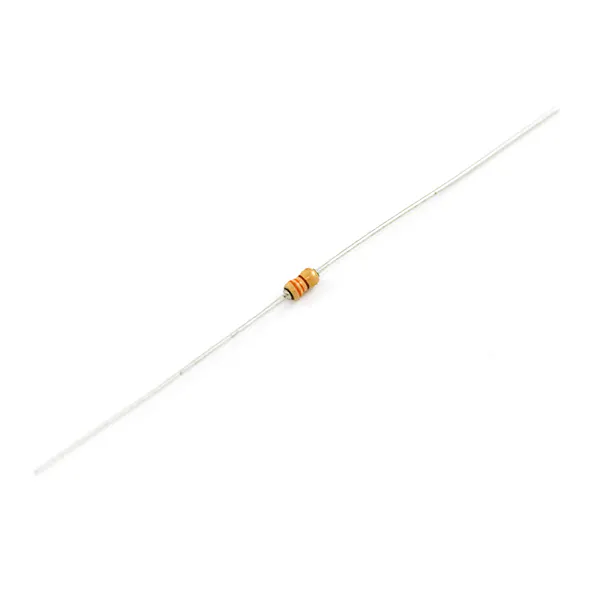Resistor 330 ohm I used a 330 ohm resistor pack from my parts bin. Anything from 180 ~ 470 ohm should work.
×8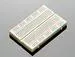Solderless Breadboard Half Size Also Available from Sparkfun
×1Jumper wires (generic)
×1

### Software apps and online servicesArduino IDE

## Schematics

### ATA8041AB 4 Digit 7 Segment LED Display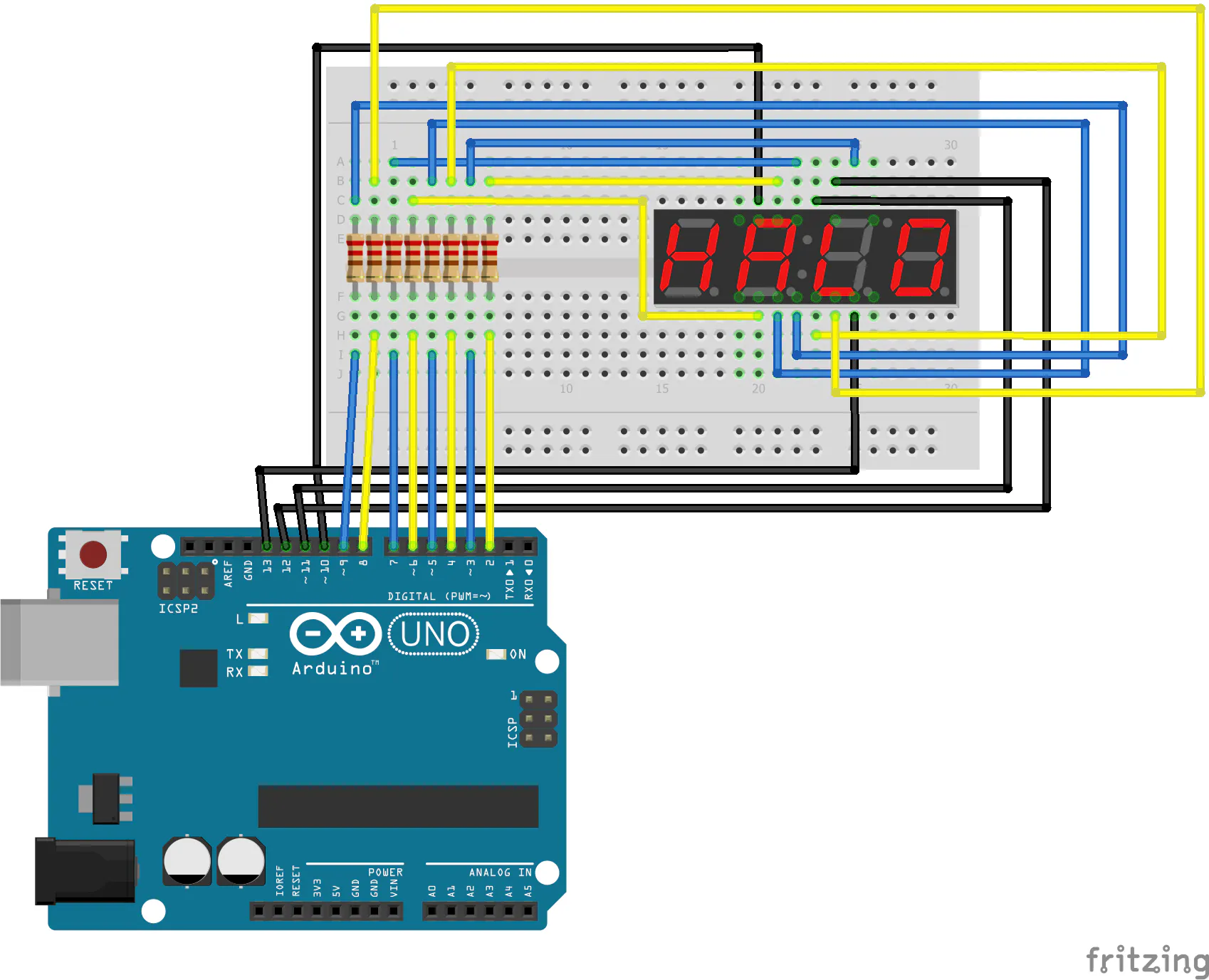## Code

### fourDigitSevenSegmentLEDcounter

Arduino
Demonstration sketch for using a Four Digit Seven Segment LED Common Cathode Display with an Arduino Compatible development board.
```/*  fourDigitSevenSegmentLEDcounter.ino by Mel Lester Jr. 2016-08-07
Derived from http://www.instructables.com/id/Arduino-4-digit-7-segment-display/
Demonstrates one way to drive the Sparkfun COM-11408 20mm 7-Segment 4-Digit Display
2017-02-26 by Mel Lester Jr. to clarify description of Display as Common Cathode

Continously displays integers from 0 to 9999

The ATA8041AB is a Common Cathode Display with seperate ground pins for each digit.
There are eight matrixed annode pins, one for each segment A-F plus a decimal point,
and four seperate grounds, one for each digit.
When a ground pin is LOW, that digit lights up.
*/
// Arduino Uno compatible pin assignments
int aPin = 2;  //         A
int bPin = 3;  //      ________
int cPin = 4;  //     |        |
int dPin = 5;  //  F  |        |  B
int ePin = 6;  //     |   G    |
int fPin = 7;  //     |________|
int gPin = 8;  //     |        |
int GND1 = 10; //     |        |
int GND2 = 11; //   E |        |   C
int GND3 = 12; //     |________|
int GND4 = 13; //
int dPnt = 9;  //         D       O dPnt
// decimal point is not used in this example code, but is included just because
int dig1 = 0;
int dig2 = 0;
int dig3 = 0;
int dig4 = 0;

int DTime = 4;

int i = 0;
long timer = millis() + 500;

void setup()
{
pinMode(aPin, OUTPUT);
pinMode(bPin, OUTPUT);
pinMode(cPin, OUTPUT);
pinMode(dPin, OUTPUT);
pinMode(ePin, OUTPUT);
pinMode(fPin, OUTPUT);
pinMode(gPin, OUTPUT);
pinMode(GND1, OUTPUT);
pinMode(GND2, OUTPUT);
pinMode(GND3, OUTPUT);
pinMode(GND4, OUTPUT);
pinMode(dPnt, OUTPUT);

Serial.begin(9600);
}

void loop()
{
// turn off all display digits
digitalWrite( GND1, HIGH);
digitalWrite( GND2, HIGH);
digitalWrite( GND3, HIGH);
digitalWrite( GND4, HIGH);

while (i <10000)
{
if (millis() > timer) { // increment counter
i++;
if (i > 9999) {
i = 0;
}
dig1 = i / 1000;
dig2 = (i - (dig1 * 1000)) / 100;
dig3 = (i - (dig1 * 1000 + dig2 * 100)) / 10;
dig4 = i % 10;
timer = millis() + 500;
}

digitalWrite( GND4, LOW);    //digit 4
pickNumber(dig4);
delay(DTime);
digitalWrite( GND4, HIGH);

digitalWrite( GND3, LOW);    //digit 3
pickNumber(dig3);
delay(DTime);
digitalWrite( GND3, HIGH);

digitalWrite( GND2, LOW);   //digit 2
pickNumber(dig2);
delay(DTime);
digitalWrite( GND2, HIGH);

digitalWrite( GND1, LOW);   //digit 1
pickNumber(dig1);
delay(DTime);
digitalWrite( GND1, HIGH);
}

} // end loop

void pickNumber(int x){
switch(x){
case 1: one(); break;
case 2: two(); break;
case 3: three(); break;
case 4: four(); break;
case 5: five(); break;
case 6: six(); break;
case 7: seven(); break;
case 8: eight(); break;
case 9: nine(); break;
default: zero(); break;
}
}

void clearLEDs()
{
digitalWrite(  2, LOW); // A
digitalWrite(  3, LOW); // B
digitalWrite(  4, LOW); // C
digitalWrite(  5, LOW); // D
digitalWrite(  6, LOW); // E
digitalWrite(  7, LOW); // F
digitalWrite(  8, LOW); // G
}

void one()
{
digitalWrite( aPin, LOW);
digitalWrite( bPin, HIGH);
digitalWrite( cPin, HIGH);
digitalWrite( dPin, LOW);
digitalWrite( ePin, LOW);
digitalWrite( fPin, LOW);
digitalWrite( gPin, LOW);
}

void two()
{
digitalWrite( aPin, HIGH);
digitalWrite( bPin, HIGH);
digitalWrite( cPin, LOW);
digitalWrite( dPin, HIGH);
digitalWrite( ePin, HIGH);
digitalWrite( fPin, LOW);
digitalWrite( gPin, HIGH);
}

void three()
{
digitalWrite( aPin, HIGH);
digitalWrite( bPin, HIGH);
digitalWrite( cPin, HIGH);
digitalWrite( dPin, HIGH);
digitalWrite( ePin, LOW);
digitalWrite( fPin, LOW);
digitalWrite( gPin, HIGH);
}

void four()
{
digitalWrite( aPin, LOW);
digitalWrite( bPin, HIGH);
digitalWrite( cPin, HIGH);
digitalWrite( dPin, LOW);
digitalWrite( ePin, LOW);
digitalWrite( fPin, HIGH);
digitalWrite( gPin, HIGH);
}

void five()
{
digitalWrite( aPin, HIGH);
digitalWrite( bPin, LOW);
digitalWrite( cPin, HIGH);
digitalWrite( dPin, HIGH);
digitalWrite( ePin, LOW);
digitalWrite( fPin, HIGH);
digitalWrite( gPin, HIGH);
}

void six()
{
digitalWrite( aPin, HIGH);
digitalWrite( bPin, LOW);
digitalWrite( cPin, HIGH);
digitalWrite( dPin, HIGH);
digitalWrite( ePin, HIGH);
digitalWrite( fPin, HIGH);
digitalWrite( gPin, HIGH);
}

void seven()
{
digitalWrite( aPin, HIGH);
digitalWrite( bPin, HIGH);
digitalWrite( cPin, HIGH);
digitalWrite( dPin, LOW);
digitalWrite( ePin, LOW);
digitalWrite( fPin, LOW);
digitalWrite( gPin, LOW);
}

void eight()
{
digitalWrite( aPin, HIGH);
digitalWrite( bPin, HIGH);
digitalWrite( cPin, HIGH);
digitalWrite( dPin, HIGH);
digitalWrite( ePin, HIGH);
digitalWrite( fPin, HIGH);
digitalWrite( gPin, HIGH);
}

void nine()
{
digitalWrite( aPin, HIGH);
digitalWrite( bPin, HIGH);
digitalWrite( cPin, HIGH);
digitalWrite( dPin, HIGH);
digitalWrite( ePin, LOW);
digitalWrite( fPin, HIGH);
digitalWrite( gPin, HIGH);
}

void zero()
{
digitalWrite( aPin, HIGH);
digitalWrite( bPin, HIGH);
digitalWrite( cPin, HIGH);
digitalWrite( dPin, HIGH);
digitalWrite( ePin, HIGH);
digitalWrite( fPin, HIGH);
digitalWrite( gPin, LOW);
}
```

## Credits

### Mel Lester Jr

5 projects • 9 followers
Long-time UNIX System Administrator and embedded software developer.